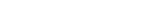MathExamle.com# Mathematical examples

Select from the list a generator that creates mathematical examples

Creates examples with addition and subtraction operations of integers, the answers are always positive

5 Variants 5 Levels
 69+22= 14+49= 30+57= 12+14= 1+94= 2+92=
Variant: 1 Level: Normal

Creates examples with multiplication and division integers

6 Variants 4 Levels
 91÷13= 96÷2= 78÷39= 20÷4= 55÷11= 0÷4= 78÷13= 70÷5=
Variant: 2 Level: Easy

Creates examples for addition or subtraction of integers by a column method

3 Variants 3 Levels
 – 395396 182878
 – 153068 120068
 – 949800 642000
Variant: 2 Level: Hard

Creates examples with a division operation, that can contain the remainder of the division

1 Variant 3 Levels
 36 7
 39 10
 16 6
 29 9
Variant: 1 Level: Easy

Creates examples with a multiplication operation of integers by a column method

1 Variant 5 Levels
 6139×2= 168×7= 9009×4= 2000×5= 7925×4= 4702×6=
Variant: 1 Level: Easy

Creates examples with a division operation of integers by a column method

1 Variant 5 Levels
 29750÷17= 2400÷24= 18732÷28= 37200÷10= 71766÷81= 96000÷40=
Variant: 1 Level: Difficult

Creates examples with operations of addition, subtraction, multiplication and division of integers, the answers are always positive

3 Variants 3 Levels
 124+92÷23+422= 274-46×2÷23= 260÷2-10×4= 292+202×2-620=
Variant: 2 Level: Normal

Creates examples with a long division of integers according to the British system

1 Variant 5 Levels
 300 9900000
 100 1500000
 128 4564864
Variant: 1 Level: Hard

Creates examples with a long division according to the British system, that can contain the remainder of the division

1 Variant 3 Levels
 10 29
 7 41
 3 29
 8 32
Variant: 1 Level: Easy

### Conversant

Creates examples with addition and subtraction operations of integers, the answers and intermediate results can be negative

4 Variants 5 Levels
 19+(-5+6)= -10-(17-11)= -8-(1+4)= -10-(-3-6)=
Variant: 3 Level: Normal

### Partitive

Creates examples with addition and subtraction operations of decimals, the answers are always positive

3 Variants 4 Levels
 99-34-49= 46.1+64.8-85.4= 83.7-19.8-36.9= 59.3-57.6+96.1=
Variant: 2 Level: Medium

Creates examples with a division operation of decimals by a column method

1 Variant 3 Levels
 23 9.2
 3.6 7.2
 30.6 8.5
 0.5 1
Variant: 1 Level: Easy

Creates examples with multiplication and division of decimals

5 Variants 4 Levels
 0.736×20= 2.6×15.12= 2.5×5.904= 1.28×3.125=
Variant: 1 Level: Difficult

### Numerator

Creates examples with addition and subtraction of fractions, the answers are always positive

3 Variants 3 Levels
 39 – 5 4 – 1 7 = 5 5 75
 37 – 13 + 50 = 8 8 77
Variant: 2 Level: Normal

Creates examples with a long division of decimals according to the British system

1 Variant 3 Levels
 9.216 63.36
 0.01 0.048
 3.125 9
Variant: 1 Level: Difficult

Creates examples with operations of addition, subtraction, multiplication and division of integers, the answers can be negative

3 Variants 3 Levels
 65×18-700= -262×5+360= 655-4×403= 503-300÷100=
Variant: 1 Level: Normal

Creates examples with operations of multiplication and division of negative and positive integers

4 Variants 4 Levels
 -3960÷88= -319×610= -125×1800= -625×(-512)=
Variant: 1 Level: Hard

Creates examples with addition and subtraction operations of decimals, the answers can be negative

3 Variants 4 Levels
 543.668-193.87-949.798= -570.204+0+473.009=
Variant: 2 Level: Hard

Creates examples with a division operation of integers, the answers may contain a decimal point

1 Variant 3 Levels
 73÷25= 36÷3= 9÷6= 99÷12= 71÷5= 50÷20=
Variant: 1 Level: Normal

Creates examples with operations of addition, subtraction, multiplication and division of decimals, the answers are always positive

3 Variants 4 Levels
 200+0.625×8+10= 100.006-453.6÷672÷7.5=
Variant: A Level: Hard

Creates examples with operations of addition, subtraction, multiplication and division of decimals, the answers can be negative

3 Variants 4 Levels
 -21.28+41.6÷1.25= -2.88×0.25-3.2÷12.8=
Variant: A Level: Normal

### Denominator

Creates examples with addition and subtraction of fractions, the answers can be negative

3 Variants 3 Levels
 10 37 + 78 153 – 28 267 = 209 209 451
Variant: 2 Level: Hard

Creates examples with multiplication and division of fractions

3 Variants 3 Levels
 23 ÷ 9 1 ÷ 7 = 5 5 10
 4 ÷ 2 × 2 1 = 5 5 2
Variant: 2 Level: Easy

Creates expressions with fractions which need to reduce

1 Variant 3 Levels
 2024 = 46
 10782 = 1422
Variant: 1 Level: Hard

Creates examples with operations of addition, subtraction, multiplication and division of fractions, the answers are always positive

2 Variants 3 Levels
 7 2 × ( 9 4 – 46 ) = 3 5 5
 5 7 ÷ 4 – 1 = 9 7 9
Variant: A Level: Easy

Creates examples with a long division of integers according to the British system, the answers may contain a decimal point

1 Variant 3 Levels
 21÷10= 90÷5= 88÷2= 23÷50= 88÷25= 40÷5=
Variant: 1 Level: Normal

Creates examples with operations of addition, subtraction, multiplication and division of fractions, the answers can be negative

2 Variants 3 Levels
 – 48 1 – 26 5 × 5 = 8 8 71
 – 7 × 26 34 + 22 11 = 8 49 21
Variant: 1 Level: Normal